# 七、提取图像特征和描述符

• 特征检测器与描述符，用于从图像中提取特征/描述符
• Harris 角点检测器和 Harris 角点特征在图像匹配中的应用（与 scikit 图像）
• 带有 LoG、DoG 和 DoH 的水滴探测器（带有scikit-image
• 方向梯度特征直方图的提取
• SIFT、ORB 和简短特征及其在图像匹配中的应用
• 类 Haar 特征及其在人脸检测中的应用

# 使用 scikit 图像

image = imread('../images/chess_football.png') # RGB imageimage_gray = rgb2gray(image)coordinates = corner_harris(image_gray, k =0.001)image[coordinates>0.01*coordinates.max()]=[255,0,0,255]pylab.figure(figsize=(20,10))pylab.imshow(image), pylab.axis('off'), pylab.show()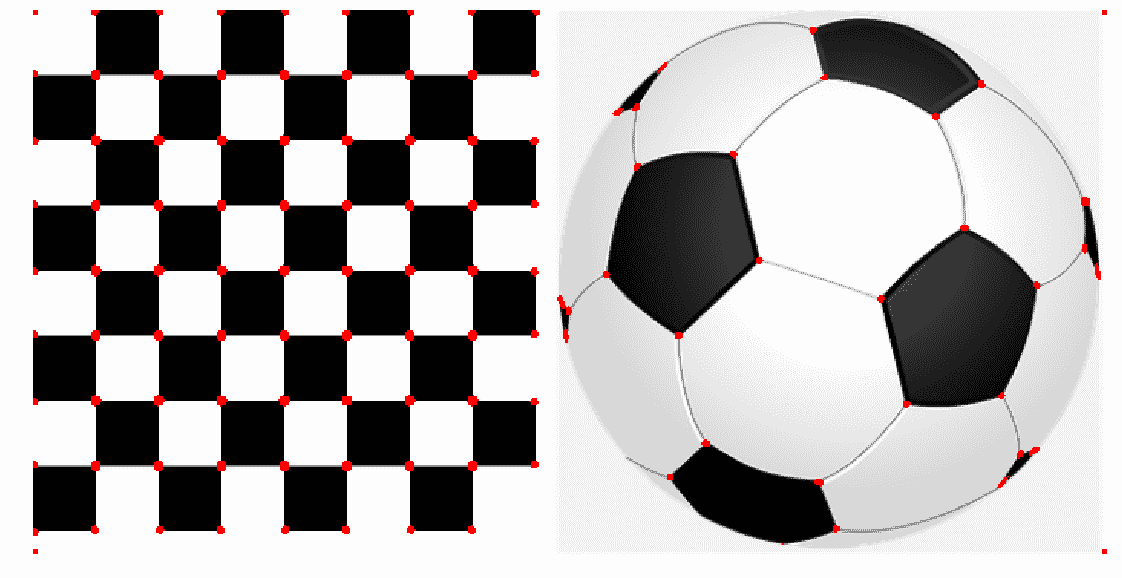# 亚像素精度

image = imread('../images/pyramids2.jpg')
image_gray = rgb2gray(image)
coordinates = corner_harris(image_gray, k =0.001)
coordinates[coordinates > 0.03*coordinates.max()] = 255 # threshold for an optimal value, depends on the image
corner_coordinates = corner_peaks(coordinates)
coordinates_subpix = corner_subpix(image_gray, corner_coordinates, window_size=11)
pylab.figure(figsize=(20,20))
pylab.subplot(211), pylab.imshow(coordinates, cmap='inferno')
pylab.plot(coordinates_subpix[:, 1], coordinates_subpix[:, 0], 'r.', markersize=5, label='subpixel')
pylab.legend(prop={'size': 20}), pylab.axis('off')
pylab.subplot(212), pylab.imshow(image, interpolation='nearest')
pylab.plot(corner_coordinates[:, 1], corner_coordinates[:, 0], 'bo', markersize=5)
pylab.plot(coordinates_subpix[:, 1], coordinates_subpix[:, 0], 'r+', markersize=10), pylab.axis('off')
pylab.tight_layout(), pylab.show()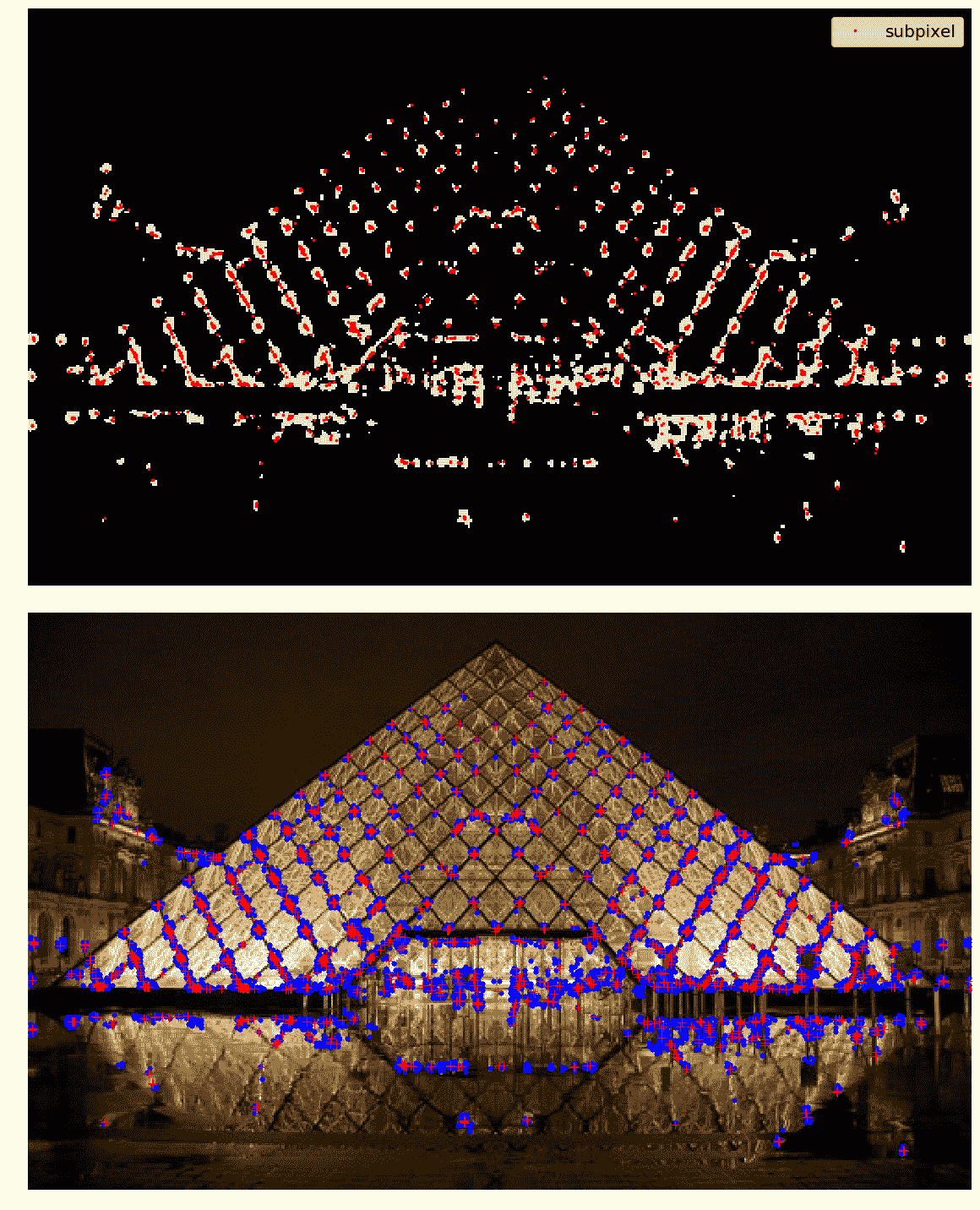# 一种应用——图像匹配

• 计算感兴趣的点（例如，使用 Harris 角点检测器的角点）
• 考虑每个关键点周围的区域（窗口）
• 从区域中，为每个图像的每个关键点计算一个局部特征描述符，并进行规格化
• 匹配两幅图像中计算的局部描述符（例如，使用欧几里德距离）

# 基于 RANSAC 算法和 Harris 角点特征的鲁棒图像匹配

1. 首先，我们将计算两幅图像中的兴趣点或 Harris 角点。
2. 将考虑点周围的小空间，然后使用平方差的加权和计算点之间的对应关系。此度量不是非常健壮，仅在视点发生轻微变化时可用。
3. 一旦找到对应，将获得一组源坐标和相应的目标坐标；它们用于估计两幅图像之间的几何变换。
4. 用坐标简单地估计参数是不够的，许多对应关系可能是错误的。

5. 使用随机样本一致性（RANSAC）算法对参数进行稳健估计，首先将点分类为inliersoutliers，然后在忽略outliers的情况下将模型拟合到inliers，以找到与仿射变换一致的匹配。

temple = rgb2gray(img_as_float(imread('../images/temple.jpg')))
image_original = np.zeros(list(temple.shape) + )
image_original[..., 0] = temple
image_original = rescale_intensity(image_original)
image_original_gray = rgb2gray(image_original)
affine_trans  = AffineTransform(scale=(0.8, 0.9), rotation=0.1, translation=(120, -20))
image_warped  = warp(image_original, affine_trans .inverse, output_shape=image_original.shape)
image_warped_gray = rgb2gray(image_warped)



coordinates = corner_harris(image_original_gray)
coordinates[coordinates > 0.01*coordinates.max()] = 1
coordinates_original = corner_peaks(coordinates, threshold_rel=0.0001, min_distance=5)
coordinates = corner_harris(image_warped_gray)
coordinates[coordinates > 0.01*coordinates.max()] = 1
coordinates_warped = corner_peaks(coordinates, threshold_rel=0.0001, min_distance=5)


coordinates_original_subpix = corner_subpix(image_original_gray, coordinates_original, window_size=9)
coordinates_warped_subpix = corner_subpix(image_warped_gray, coordinates_warped, window_size=9)

def gaussian_weights(window_ext, sigma=1):
y, x = np.mgrid[-window_ext:window_ext+1, -window_ext:window_ext+1]
g_w = np.zeros(y.shape, dtype = np.double)
g_w[:] = np.exp(-0.5 * (x**2 / sigma**2 + y**2 / sigma**2))
g_w /= 2 * np.pi * sigma * sigma
return g_w


def match_corner(coordinates, window_ext=3):
row, col = np.round(coordinates).astype(np.intp)
window_original = image_original[row-window_ext:row+window_ext+1, col-window_ext:col+window_ext+1, :]
weights = gaussian_weights(window_ext, 3)
weights = np.dstack((weights, weights, weights))
SSDs = []
for coord_row, coord_col in coordinates_warped:
window_warped = image_warped[coord_row-window_ext:coord_row+window_ext+1,
coord_col-window_ext:coord_col+window_ext+1, :]
if window_original.shape == window_warped.shape:
SSD = np.sum(weights * (window_original - window_warped)**2)
SSDs.append(SSD)
min_idx = np.argmin(SSDs) if len(SSDs) > 0 else -1
return coordinates_warped_subpix[min_idx] if min_idx >= 0 else [None]


source, destination = [], []
for coordinates in coordinates_original_subpix:
coordinates1 = match_corner(coordinates)
if any(coordinates1) and len(coordinates1) > 0 and not all(np.isnan(coordinates1)):
source.append(coordinates)
destination.append(coordinates1)
source = np.array(source)
destination = np.array(destination)


model = AffineTransform()
model.estimate(source, destination)


model_robust, inliers = ransac((source, destination), AffineTransform, min_samples=3, residual_threshold=2, max_trials=100)
outliers = inliers == False


print(affine_trans.scale, affine_trans.translation, affine_trans.rotation)
# (0.8, 0.9) [ 120\. -20.] 0.09999999999999999
print(model.scale, model.translation, model.rotation)
# (0.8982412101241938, 0.8072777593937368) [ -20.45123966 114.92297156] -0.10225420334222493
print(model_robust.scale, model_robust.translation, model_robust.rotation)
# (0.9001524425730119, 0.8000362790749188) [ -19.87491292 119.83016533] -0.09990858564132575


fig, axes = pylab.subplots(nrows=2, ncols=1, figsize=(20,15))
pylab.gray()
inlier_idxs = np.nonzero(inliers)
plot_matches(axes, image_original_gray, image_warped_gray, source, destination, np.column_stack((inlier_idxs, inlier_idxs)), matches_color='b')
axes.axis('off'), axes.set_title('Correct correspondences', size=20)
outlier_idxs = np.nonzero(outliers)
plot_matches(axes, image_original_gray, image_warped_gray, source, destination, np.column_stack((outlier_idxs, outlier_idxs)), matches_color='row')
axes.axis('off'), axes.set_title('Faulty correspondences', size=20)
fig.tight_layout(), pylab.show()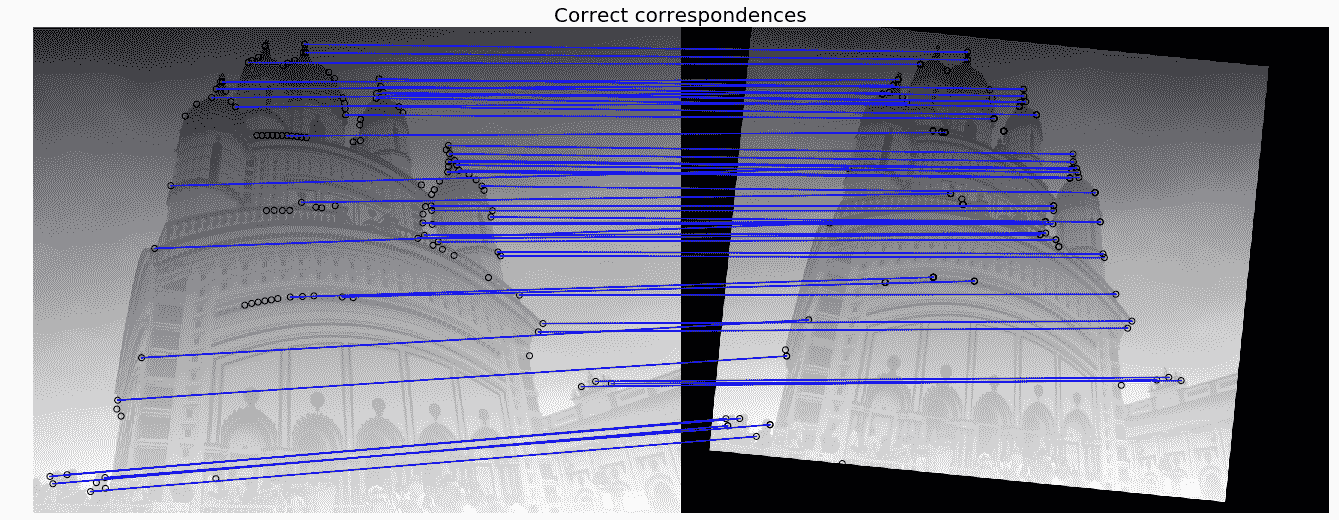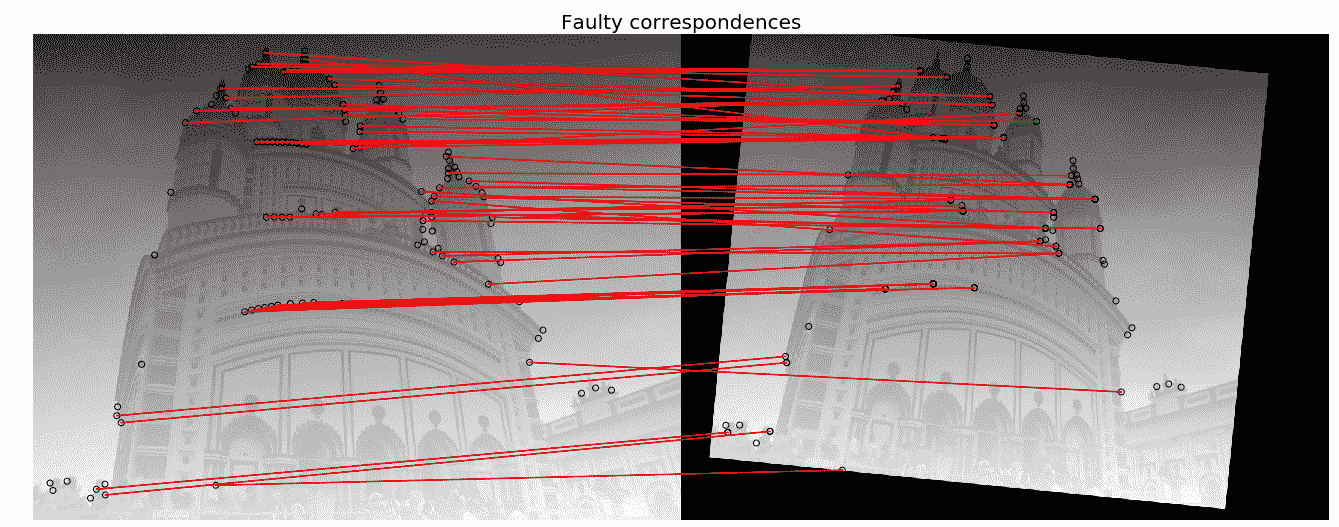# 高斯差分（DoG）

LoG 方法近似于 DoG 方法，因此速度更快。图像用增加的σ值进行平滑（使用高斯），两个连续平滑图像之间的差异叠加在一个立方体中。这种方法再次检测黑暗背景上的亮斑。它比日志更快，但精确度较低，尽管较大的斑点检测仍然昂贵。

# 黑森行列式（DoH）

DoH 方法是所有这些方法中最快的。它通过计算图像 Hessian 行列式矩阵的最大值来检测斑点。水滴的大小对检测速度没有任何影响。该方法既能检测出暗背景上的亮斑，又能检测出亮背景上的暗斑，但不能准确地检测出小斑。

from numpy import sqrt
from skimage.feature import blob_dog, blob_log, blob_doh

im_gray = rgb2gray(im)
log_blobs = blob_log(im_gray, max_sigma=30, num_sigma=10, threshold=.1)
log_blobs[:, 2] = sqrt(2) * log_blobs[:, 2] # Compute radius in the 3rd column
dog_blobs = blob_dog(im_gray, max_sigma=30, threshold=0.1)
dog_blobs[:, 2] = sqrt(2) * dog_blobs[:, 2]
doh_blobs = blob_doh(im_gray, max_sigma=30, threshold=0.005)
list_blobs = [log_blobs, dog_blobs, doh_blobs]
color, titles = ['yellow', 'lime', 'red'], ['Laplacian of Gaussian', 'Difference of Gaussian', 'Determinant of Hessian']
sequence = zip(list_blobs, colors, titles)
fig, axes = pylab.subplots(2, 2, figsize=(20, 20), sharex=True, sharey=True)
axes = axes.ravel()
axes.imshow(im, interpolation='nearest')
axes.set_title('original image', size=30), axes.set_axis_off()
for idx, (blobs, color, title) in enumerate(sequence):
axes[idx+1].imshow(im, interpolation='nearest')
axes[idx+1].set_title('Blobs with ' + title, size=30)
for blob in blobs:
y, x, row = blob
col = pylab.Circle((x, y), row, color=color, linewidth=2, fill=False)
pylab.tight_layout(), pylab.show()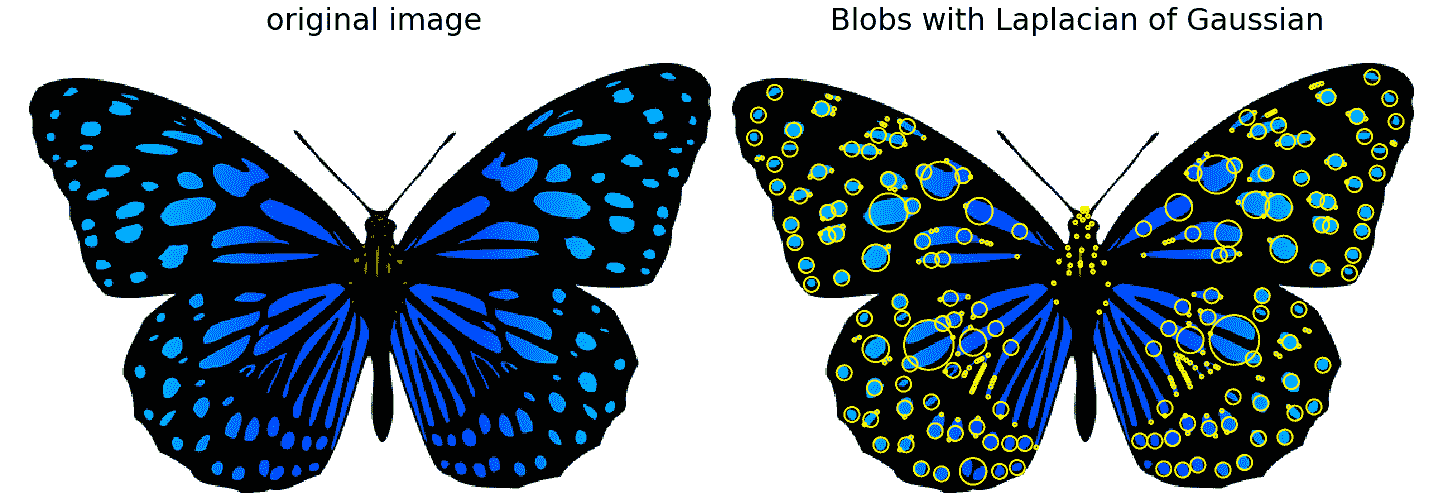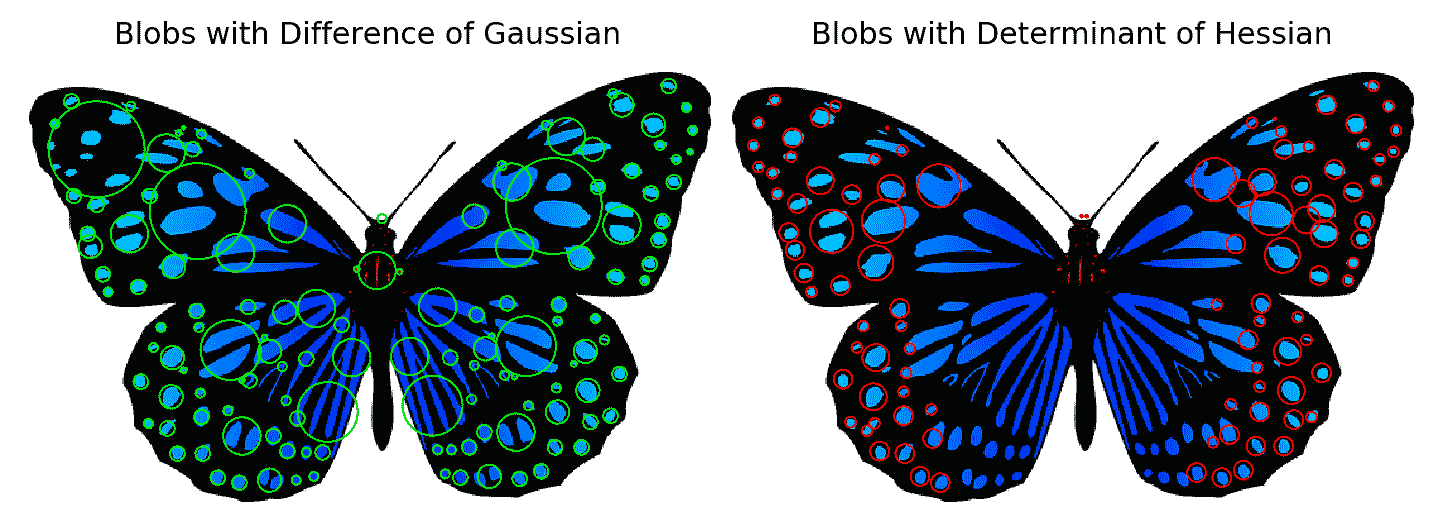# 计算 HOG 描述符的算法

1. 如果愿意，可以对图像进行全局规格化
2. 计算水平和垂直梯度图像
3. 计算梯度直方图
4. 跨块标准化
5. 展平为特征描述符向量

HOG 描述符是使用该算法最终得到的归一化块描述符

# 使用 scikit 图像计算 HOG 描述符

from skimage.feature import hogfrom skimage import exposureimage = rgb2gray(imread('../images/cameraman.jpg'))fd, hog_image = hog(image, orientations=8, pixels_per_cell=(16, 16), cells_per_block=(1, 1), visualize=True) print(image.shape, len(fd))# ((256L, 256L), 2048)fig, (axes1, axes2) = pylab.subplots(1, 2, figsize=(15, 10), sharex=True, sharey=True)axes1.axis('off'), axes1.imshow(image, cmap=pylab.cm.gray), axes1.set_title('Input image')


hog_image_rescaled = exposure.rescale_intensity(hog_image, in_range=(0, 10))axes2.axis('off'), ...


# SIFT 描述符的计算算法

• 尺度空间极值检测：搜索多个尺度和图像位置，位置和特征尺度由 DoG 检测器给出
• 关键点定位：基于稳定性度量选择关键点，通过消除低对比度和边缘关键点，仅保留强兴趣点
• 方向分配：计算每个关键点区域的最佳方向，有助于匹配的稳定性
• 关键点描述符计算：使用选定比例和旋转的局部图像梯度来描述每个关键点区域

# 使用 opencv 和 opencv contrib

# make sure the opencv version is 3.3.0 with
# pip install opencv-python==3.3.0.10 opencv-contrib-python==3.3.0.10

import cv2
print(cv2.__version__)
# 3.3.0

gray= cv2.cvtColor(img,cv2.COLOR_BGR2GRAY)

sift = cv2.xfeatures2d.SIFT_create()
kp = sift.detect(gray,None) # detect SIFT keypoints

img = cv2.drawKeypoints(img,kp, None, flags=cv2.DRAW_MATCHES_FLAGS_DRAW_RICH_KEYPOINTS)
cv2.imshow("Image", img);
cv2.imwrite('me5_keypoints.jpg',img)

kp, des = sift.detectAndCompute(gray,None) # compute the SIFT descriptor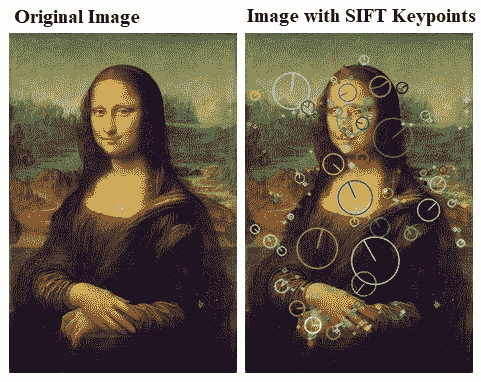# 使用简短的二进制描述符将图像与 scikit 图像匹配

from skimage import transform as transform
from skimage.feature import (match_descriptors, corner_peaks, corner_harris, plot_matches, BRIEF)
affine_trans = transform.AffineTransform(scale=(1.2, 1.2), translation=(0,-100))
img2 = transform.warp(img1, affine_trans)
img3 = transform.rotate(img1, 25)
coords1, coords2, coords3 = corner_harris(img1), corner_harris(img2), corner_harris(img3)
coords1[coords1 > 0.01*coords1.max()] = 1
coords2[coords2 > 0.01*coords2.max()] = 1
coords3[coords3 > 0.01*coords3.max()] = 1
keypoints1 = corner_peaks(coords1, min_distance=5)
keypoints2 = corner_peaks(coords2, min_distance=5)
keypoints3 = corner_peaks(coords3, min_distance=5)
extractor = BRIEF()
extractor.extract(img1, keypoints1)
extractor.extract(img2, keypoints2)
extractor.extract(img3, keypoints3)
matches12 = match_descriptors(descriptors1, descriptors2, cross_check=True)
matches13 = match_descriptors(descriptors1, descriptors3, cross_check=True)
fig, axes = pylab.subplots(nrows=2, ncols=1, figsize=(20,20))
pylab.gray(), plot_matches(axes, img1, img2, keypoints1, keypoints2, matches12)
axes.axis('off'), axes.set_title("Original Image vs. Transformed Image")
plot_matches(axes, img1, img3, keypoints1, keypoints3, matches13)
axes.axis('off'), axes.set_title("Original Image vs. Transformed Image"), pylab.show()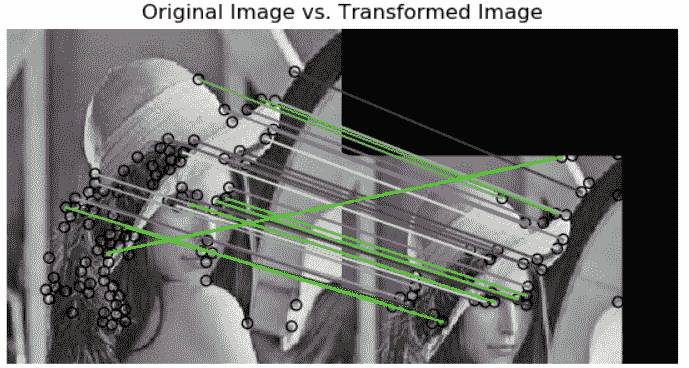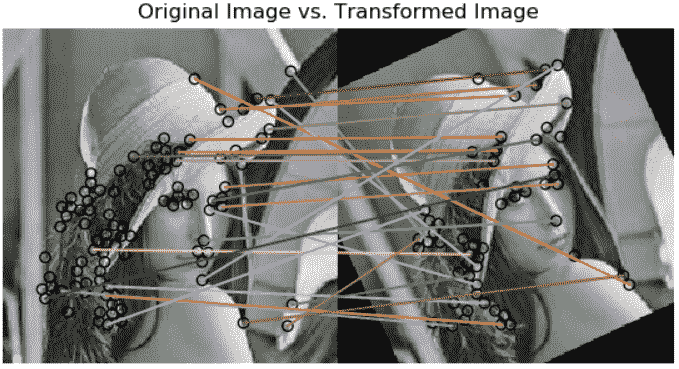# 使用 scikit 图像匹配 ORB 特征检测器和二进制描述符

from skimage import transform as transformfrom skimage.feature import (match_descriptors, ORB, plot_matches)img1 = rgb2gray(imread('../images/me5.jpg'))img2 = transform.rotate(img1, 180)affine_trans = transform.AffineTransform(scale=(1.3, 1.1), ...


# 使用蛮力匹配 python opencv 与 ORB 特性匹配

img1 = cv2.imread('../images/books.png',0) # queryImage
# Create a ORB detector object
orb = cv2.ORB_create()
# find the keypoints and descriptors
kp1, des1 = orb.detectAndCompute(img1,None)
kp2, des2 = orb.detectAndCompute(img2,None)
# create a BFMatcher object
bf = cv2.BFMatcher(cv2.NORM_HAMMING, crossCheck=True)
# Match descriptors.
matches = bf.match(des1, des2)
# Sort them in the order of their distance.
matches = sorted(matches, key = lambda x:x.distance)
# Draw first 20 matches.
img3 = cv2.drawMatches(img1,kp1,img2,kp2,matches[:20], None, flags=2)
pylab.figure(figsize=(20,10)), pylab.imshow(img3), pylab.show()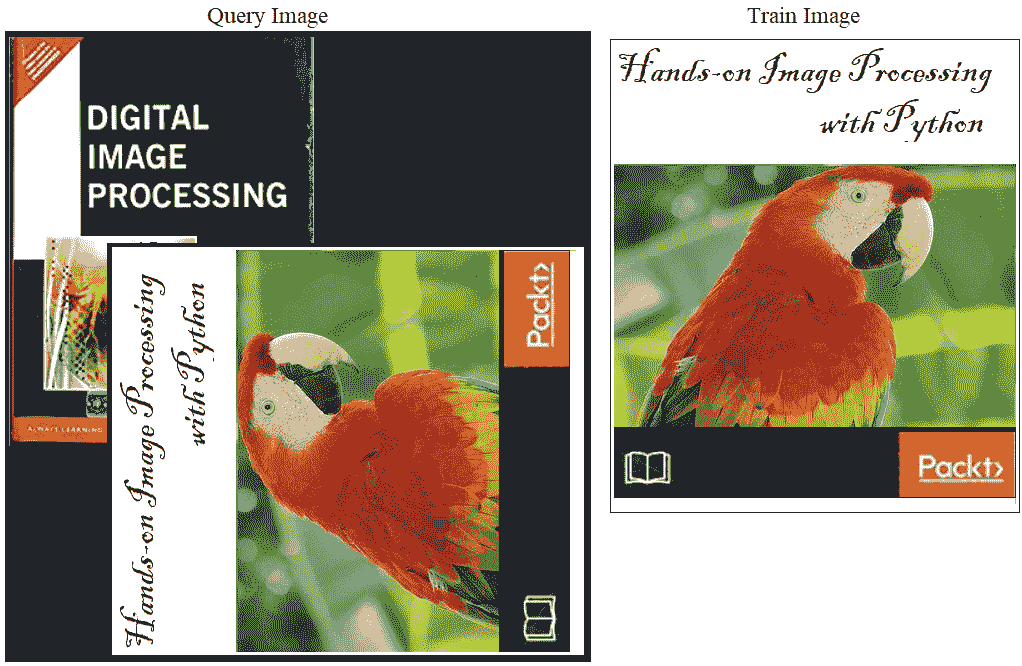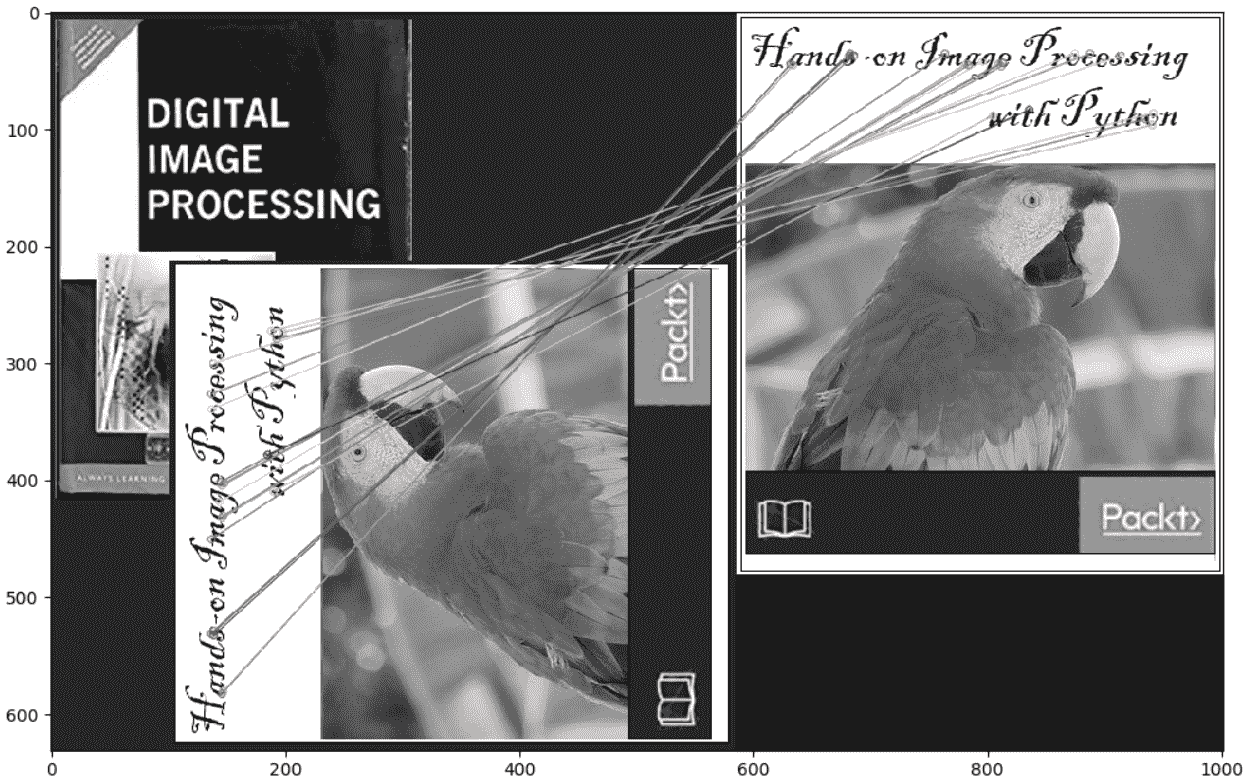# 使用 SIFT 描述符的蛮力匹配和使用 OpenCV 的比率测试

# make sure the opencv version is 3.3.0 with# pip install opencv-python==3.3.0.10 ...


# 类哈尔特征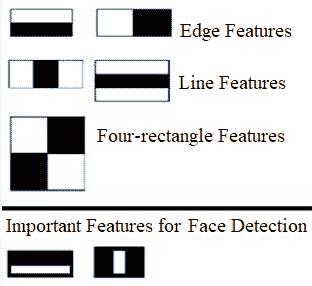# 带有 scikit 图像的类 Haar 特征描述符

from skimage.feature import haar_like_feature_coordfrom skimage.feature import draw_haar_like_featureimages = [np.zeros((2, 2)), np.zeros((2, 2)), np.zeros((3, 3)),np.zeros((3, 3)), np.zeros((2, 2))]feature_types = ['type-2-x', 'type-2-y', 'type-3-x', ...


# 基于预训练分类器和 Haar 级联特征的 OpenCV 人脸/眼睛检测

OpenCV 配有一个培训师和一个检测器。在本节中，我们将演示使用预先训练的人脸、眼睛、微笑等分类器进行检测（跳过训练模型）。OpenCV 已经包含了许多已经训练过的模型；我们将使用它们，而不是从头开始训练分类器。这些预先训练好的分类器被序列化为 XML 文件，并附带 OpenCV 安装（可以在opencv/data/haarcascades/文件夹中找到）

# 问题

1. 使用cv2实现亚像素精度的 Harris 角点检测器。
2. 使用cv2使用几个不同的预先训练好的 Haar Cascade 分类器，尝试从图像中检测多张人脸。
3. 使用基于 FLANN 的近似最近邻匹配器代替BFMatcher将图像与具有cv2的书籍进行匹配。
4. 计算 SURF 关键点并使用它们与cv2进行图像匹配